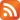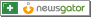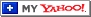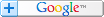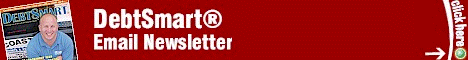Web DebtSmart.comTuesday, May 18, 2021
 Amortized Loans by Scott Bilker
 Scott Bilker is the author of the best-selling books, Talk Your Way Out of Credit Card Debt, Credit Card and Debt Management, and How to be more Credit Card and Debt Smart. He's also the founder of DebtSmart.com. More about Scott Bilker and DebtSmart can be found in the online media kit.Scott,

I would like to know what the formula is for an amortized loan. How exactly do they calculate each payment? I suspect that all the interest for the loan term (i.e., 30 years, 15 years, etc.) is loaded up front in some way, but I would like to know exactly HOW? I want to teach my teenagers about this. I think it's very important for everyone to know.

If everyone understood these things that affect us all, there would be fewer people getting into financial trouble.
Thank you.
--Maria

Maria,Thanks for writing! That's a great question and one I will truly enjoy answering! My background is in Engineering and math and I do love math. It's a good thing too, because doing the math has helped me make the right buying decisions and avoid being tricked countless times.

However, I must warn that the explanation of the formula to calculate loans is quite involved so be ready to get an Algebra refresher.

First thing to address is your suspicion that loans are "loaded up front." You can think of it this way but it's better think in terms of periodic (monthly), discrete transactions. The way all loans work is quite simple.

At some periodic time, usually monthly, the amount owed is multiplied by the periodic interest rate. That amount is then added to the original amount owed and any payments are subtracted. That's it. The mathematical formula is: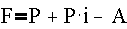Equation #1

Where:
F = Future value of an annuity (unpaid balance)
P = Principal value
i = Periodic interest (APR divided by 12)
n = Number of periods (months for most loans)
A = Periodic payment (monthly payment for most loans)

For example:
Say you have a \$10,000 loan at 12% APR. At the end of the first month the bank is going to calculate the interest by multiplying \$10,000 by the periodic rate (monthly rate). The monthly rate is 1% because 12x1%=12%APR. So the interest charge is 1% of \$10,000 or \$100. That amount is added to the balance so the new balance is \$10,100 or: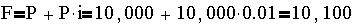From this amount the bank will subtract your payments (A). It is apparent that you must pay more than \$100 or you'll be in a situation where the balance will never decrease. If you pay \$100 then that would be subtracted from the new balance and you're back to owing \$10,000. At that rate the loan will never be paid off.

To pay off the loan in a specific number of periods (months) you must make a certain payment that's greater than the periodic interest charge.

The formula to find the monthly payment (A) to pay off a loan in n periods is: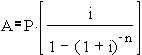Equation #2

The formula to find the future balance (unpaid balance) of a loan is: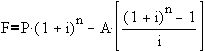Equation #3

Notice that if F=0 in equation #3, and we solve for A, we get equation #2.

Granted, equations 2 and 3 don't look as simple as equation 1, but keep in mind that they are based on that simple equation 1.

Let's use these formulas in the first example. Say you want to pay off that \$10,000, 12% APR loan in exactly 5 years. What payment do you have to make to do this?

Let's list the variables:
P = \$10,000
i = 1% (APR divided by 12)
n = 60 months (5 years)
A = Periodic payment (monthly payment). This is what we're looking to find.

To find the monthly payment use equation #2 as follows: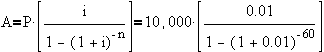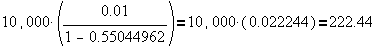The monthly payment for this loan is \$222.44. Now let's say you want to find out how much you owe on the loan after making 15 monthly payment.

Let's list the variables:
P = \$10,000
i = 1% (APR divided by 12)
n = 15 months
A = \$222.44

Using equation #3 as follows: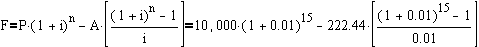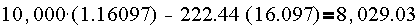After 15 payments of \$222.44 the unpaid balance remaining is \$8,029.03.

Conclusion:
This article discussed how to use the formulas associated with finding the payment and amortization of annuities (loans). I'll save the derivation of these functions for another article.

Hope that helps!

Talk to you later.

Regards,
Scott

--End--

 Subscribe FREE and start finding new ways to save money and pay off your debt. "The DebtSmart Email Newsletter is packed with cutting-edge strategies for solving credit problems. I highly recommend it."--Gerri Detweiler, radio host and author of The Ultimate Credit HandbookDEBTSMART MEDIA MENTIONSNBC 10 News: Money King Secrets CN8:Art Fennell Reports CNN: CNN Newsroom CNN: American Morning ABC: Action News CNN/fn: Your Money

 Subscribe to the DebtSmart® RSS Feed